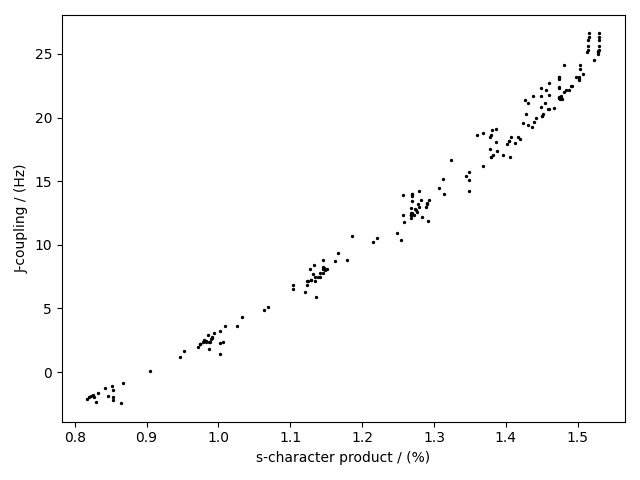Scatter, 0D{1,1} dataset¶

We start with a 0D{1,1} correlated dataset, that is, a dataset without a coordinate grid. A 0D{1,1} dataset has no dimensions, d = 0, and two single-component dependent variables. In the following example 1, the two correlated dependent variables are the $$^{29}\text{Si}$$ - $$^{29}\text{Si}$$ nuclear spin couplings, $$^2J$$, across a Si-O-Si linkage, and the s-character product on the O and two Si along the Si-O bond across the Si-O-Si linkage.

Let’s import the dataset.

import csdmpy as cp

filename = "https://osu.box.com/shared/static/h1nxth6gs94fthfmvip5vchp3zh4zd6o.csdf"

Since the dataset has no dimensions, the value of the dimensions attribute of the CSDM class is an empty tuple,

Out:

[]

The dependent_variables attribute, however, holds two dependent-variable objects. The data structure from the two dependent variables is

print(zero_d_dataset.dependent_variables.data_structure)

Out:

{
"type": "internal",
"name": "Gaussian computed J-couplings",
"unit": "Hz",
"quantity_name": "frequency",
"numeric_type": "float32",
"quantity_type": "scalar",
"component_labels": [
"J-coupling"
],
"components": [
[
"-1.87378, -1.42918, ..., 25.1742, 26.0608"
]
]
}

and

print(zero_d_dataset.dependent_variables.data_structure)

Out:

{
"type": "internal",
"name": "product of s-characters",
"unit": "%",
"numeric_type": "float32",
"quantity_type": "scalar",
"component_labels": [
"s-character product"
],
"components": [
[
"0.8457453, 0.8534185, ..., 1.5277092, 1.5289451"
]
]
}

respectively.

Visualizing the dataset

The correlation plot of the dependent-variables from the dataset is shown below.Citation

1

Srivastava DJ, Florian P, Baltisberger JH, Grandinetti PJ. Correlating geminal couplings to structure in framework silicates. Phys Chem Chem Phys. 2018;20:562–571. DOI:10.1039/C7CP06486A

Total running time of the script: ( 0 minutes 1.565 seconds)

Gallery generated by Sphinx-Gallery